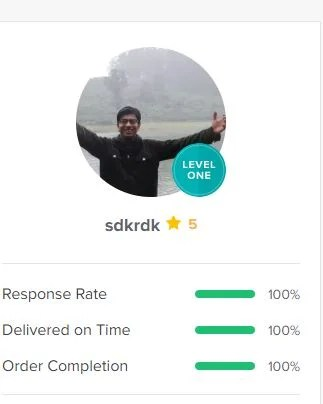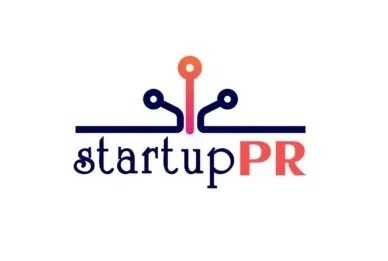# Kids and Mathematics. Here are the platforms in India that can decode the puzzle for your kidsSchools have been closed since March 2020  last year. This has put more pressure on parents for their child’s education. Mathematics is a subject most of the students struggle with, so it is essential to build a strong foundation in the child’s learning years so that they understand the subject better. Mathematical concepts are something that we use every day without even realizing it- it helps us in reasoning and enhancing problem-solving skills. Thus, children must have a good understanding of the subject.

Here are 5 platforms that can decode the puzzle for your kids:

1. British Youth International College

This UK-based platform teaches kids maths through abacus. Abacus focuses on the skills of memory, concentration, analysis, visualization, and control. This online platform uses an instructor-led approach that guides students at every step. The highly qualified teachers at BYITC provide in-depth tutoring and feedback to each student, giving them the space to improvise at each step. They cover all mathematical concepts of addition, subtraction, multiplication division, and even decimal calculations.

1. KooBits

KooBits uses animated videos and reward systems to make mathematics fun for children. It teaches maths to children of ages between 6-12 years. It closely follows the Singapore method of mathematics which is recognized globally and is the most effective method for building a strong foundation in mathematics. It’s interactive virtual classes that break down mathematical concepts to make learning easy for the child.

1. Mindspark

Mindspark is a platform that is aligned with the school syllabus of BBSC, ICSE, and IGCSE and provides maths classes from class 1 to class 10. It makes mathematics learning fun by giving students solving challenging mathematics questions that help boost the students’ confidence. Detailed feedback is given on every response and a report is prepared for the parent to gauge their child’s development. They teach all mathematical concepts like Algebra, Geometry, Measurement, Addition, Subtraction Multiplication, etc.

1. Mathspace

Mathspace is an adaptive math platform. Two teachers are assigned to each student and one of them is available 24/7. They guide students step by step so that students are not anxious about making mistakes. By giving step-by-step hints, problem-solving becomes easier and concepts become more clear. With 14000+ videos, 60,000+ interactive questions, and 600+ digital textbooks, learning is fun and more interactive for the students.

1. Cuemaths

Cuemaths provides maths lessons from KG to class 12. For learners till class 2, Cuemaths provide number sense, counting, and basic arithmetic and from classes 9-12 they provide lessons on Algebra, Geometry, Trigonometry, Vectors, and Calculus. Their rigorous training and constant monitoring and workshops help students learn mathematics in a fun way.Posted on July 21, 2021July 21, 2021Categories Startups